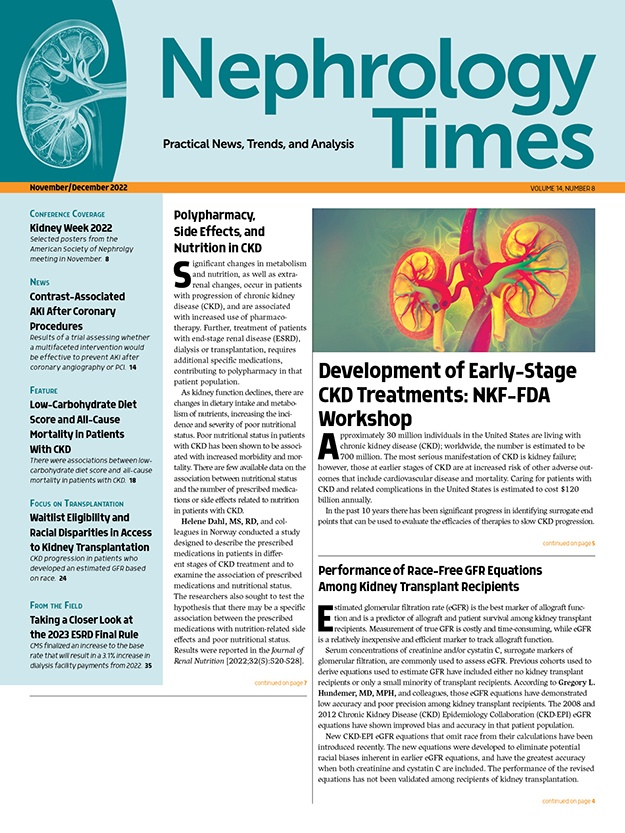# Performance of Race-Free GFR Equations Among Kidney Transplant Recipients

By Victoria Socha - Last Updated: November 29, 2022Estimated glomerular filtration rate (eGFR) is the best marker of allograft function and is a predictor of  allograft and patient survival among kidney transplant recipients. Measurement of true GFR is costly and time-consuming, while eGFR is a relatively inexpensive and efficient marker to track allograft function.

Serum concentrations of creatinine and/or cystatin C, surrogate markers of glomerular filtration, are commonly used to assess eGFR. Previous cohorts used to derive equations used to estimate GFR have included either no kidney transplant recipients or only a small minority of transplant recipients. According to Gregory L. Hundemer, MD, MPH, and colleague those eGFR equations have demonstrated low accuracy and poor precision among kidney transplant recipients. The 2008 and 2012 Chronic Kidney Disease (CKD) Epidemiology Collaboration (CKD-EPI) eGFR equations have shown improved bias and accuracy in that patient population.

New CKD-EPI eGFR equations that omit race from their calculations have been introduced recently. The new equations were developed to eliminate potential racial biases inherent in earlier eGFR equations, and have the greatest accuracy when both creatinine and cystatin C are included. The performance of the revised equations has not been validated among recipients of kidney transplantation.

Dr. Hundemer et al conducted a cross-sectional study to validate the performance of the 2021 CKD-EPI creatinine- and creatinine/cystatin C-based eGFR estimating equations (eGFRcr and eGFRcr-cys, respectively). The researchers sought to examine the bias, precision, and accuracy of the equations along with the proportion of kidney transplant recipients classified according to CKD staging. Results were reported in the American Journal of Kidney Diseases [2022;80(4):462-472].

The study cohort included 415 kidney transplant recipients. Mean age was 54 years, and 32% (n=134) were female. Mean time since transplant was 6.9 years, 41% (n=170) had received a transplant from a living donor, and 13% (n=52) had a history of more than one kidney transplant. Mean serum creatinine and serum cystatin C concentrations were 1.62 mg/dL and 1.55 mg/L, respectively. Mean measured GFR (mGFR) was 53 mL/min/1.73 m2. Thirty-six percent, 53%, and 11% of participants were categorized as having mGFRs of ≥60, 30-59, and <30 mL/min/1.73 m2, respectively.

Among the overall study population, the median biases of the 2009 and 2021 CKD-EPI eGFRcr equations were –2.3 and –0.2 mL/min/1.73 m2, respectively. Precision was similar between the 2009 and 2012 CKD-EPI eGFRcr equations (interquartile range [IQRs] of 14.5 and 14.9 mL/min/1.73 m2, respectively). Accuracy was also similar between the 2009 and 2021 CKD-EPI equations, as demonstrated by P10/P20/P30 (32%/65%/84% vs 33%/63%/84%, respectively, root mean square error (12.9 [95% CI, 11.6-14.1] vs 13.0 [95% CI, 11.8-14.4] mL/min/1.73 m2, and mean absolute error (9.7 [95% CI, 8.9-10.5] vs 9.7 [95% CI, 8.9-10.6] mL/min/1.73 m2). The performance measures were also similar when stratified by the eGFR categories of ≥60, 30-59, and <30 mL/min/1.73 m2.

In the overall study cohort, the median biases of the 2012 and 2021 CKD-EPI eGFRcr-cys equations were –3.6 and 0.3 mL/min/1.73 m2, respectively. Regarding precision, IQRs of the 2012 and 2021 CKD-EPI eGFRcr-cys equations were 13.3 and 14.3 mL/min/1.73 m2, respectively. Accuracy was also similar between the two equations. The measures were similar when stratified by the eGFR categories of ≥60, 30-59, and <30 mL/min/1.73 m2.

Among the overall study population, the 2012 CKD-EPI eGFRcys equation had a median bias of –0.8 mL/min/1.73 m2. The precision was reduced relative to the creatinine- and creatinine/cystatin C-based equations (IQR, 18.5 mL/min/1.73 m2). Accuracy was also reduced relative to the creatinine- and creatinine/cystatin C-based equations.

Compared with the other eGFR equations, the Modification of Diet in Renal Disease (MDRD) Study equation demonstrated a relatively greater median bias (–5.0 mL/min/1.73 m2). Precision of the MDRD Study equation was similar to the other GFR equations (IQR, 14.7 mL/min/1.73m2).

There was no clear difference between the 2021 CKD-EPI eGFRcr and eGFRcr-cys equations (mean eGFR difference of –0.7; 95% CI, –18.7 to 17.4) mL/min/1.73 m2.

In analyses of proportion of correct classification of CKD by eGFR equation, the 2009 CKD-EPI eGFRcr equation correctly classified 66% of participants compared with 68% with the 2021 version equation. The 2012 CKD-EPI eGFRcr-cys equation correctly classified 64% of participants, compared with 69% correctly classified with the 2021 version. The MDRD Study equation classified a similar percentage of participants (67%); the 2021 CKD-EPI eGFRcys equation correctly classified a relatively lower percentage of participants (61%).

The researchers cited some limitations to the study, including the small sample size that may have limited the power to detect subtle differences in performance between the eGFR equations (eg, between eGFRcr and eGFRcr-cys as was shown among participants who were not kidney transplant recipients in the original study). The subset of participants with eGFR <30 mL/min/1.73 m2 was small (n=45), limiting the ability to draw and definitive conclusions regarding eGFR equation performance in that subset. Another notable limitation was the small number of patients who self-identified as Black, limiting the ability to compare differences in eGFR equation by race.

In summary, the authors said, “Among kidney transplant recipients, the 2021 race-free CKD-EPI eGFR equations perform similarly to the previous CKD-EPI equations that included race correction terms. No significant difference in performance was observed between the 2021 CKD-EPI eGFRcr and eGFRcr-cys equations in the kidney transplant population.”

Takeaway Points

1. Researchers reported results of a cross-sectional study designed to validate the 2021 race-free Chronic Kidney Disease-Epidemiology Collaboration (CKD-EPI) estimated glomerular filtration rate (eGFR) equation based on creatine alone (eGFRcr) or based on creatinine and cystatin C (eGFRcr-cys) among kidney transplant recipients.
2. The 2021 race-free CKD-EPI eGFR equations performed similarly to the previous CKD-EPI equations that included race correction terms.
3. There was no significant difference in performance between the 2021 CKD-EPI eGFRcr and the CKD-EPI eGFRcr-cys equations among the cohort of kidney transplant recipients.
Post Tags:EGFR